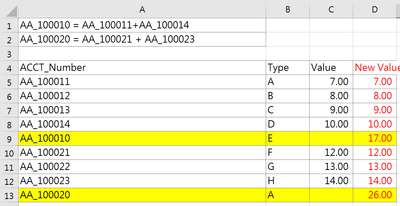cancel
Showing results for
Did you mean:Regular Visitor

## Financial analysis: How to make calculation that meets certain condition with nested if statement

Hi all,

I have a financial calculation problem to ask and would appreciate any kind help!

In the table below,  AA_100010 and AA_100020 are empty numbers. They are values from additions of other cells.

What I would like to have is creating a new column in Power Query where

IF [AACT_NUMBER]= AA_100010 Then [New Value] is AA_100011+AA_100014 which equals to 17

ELSE IF [AACT_NUMBER]= AA_100020 Then [New Value] is AA_100021 + AA_100023 which equals to 26

ELESE Return values in the [VALUE] columnThanks in advance for the help from Taiwan!

1 ACCEPTED SOLUTIONSuper User2 REPLIES 2Super UserRegular Visitor

Thank you Daniel : )